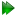Subsections

Torsionless isotropic cone equations

Figure 16.9: The torsionless isotropic cone model simulated and calculated in-frame Daeg(1) and Daeg(2) frame order matrix elements. The top row corresponds to Daeg(1) and the bottom to Daeg(2). In these plots, θX corresponds to the cone opening half-angle θ. Frame order matrix values have been calculated every 10 degrees.Figure 16.10: The torsionless isotropic cone model simulated and calculated out-of-frame Daeg(1) and Daeg(2) frame order matrix elements. The top row corresponds to Daeg(1) and the bottom to Daeg(2). In these plots, θX corresponds to the cone opening half-angle θ. Frame order matrix values have been calculated every 10 degrees.Torsionless isotropic cone rotation matrices

The torsionless rotation matrix is defined in equation 12.77c.

Torsionless isotropic cone frame order matrix

The frame order matrix isThe surface normalisation factor isTorsionless isotropic cone 1 degree frame order

The 1 degree frame order matrix with tensor rank-2 isTorsionless isotropic cone 2 degree frame order

The 2 degree frame order matrix with tensor rank-4 consists of the following elements, using Kronecker product double indices from 0 to 8After factorisation, the equations areTorsionless isotropic cone frame order matrix simulation and calculation

The frame order matrix element simulation script from Section 16.2, pagewas used to compare the implementation of equations 16.30 and 16.32 above. Frame order matrix Daeg(1) and Daeg(2) values were both simulated and calculated, both within and out of the motional eigenframe. The in-frame Daeg(1) and Daeg(2) values are shown in figure 16.9. The out-of-frame Daeg(1) and Daeg(2) values are shown in figure 16.10.

The relax user manual (PDF), created 2020-08-26.